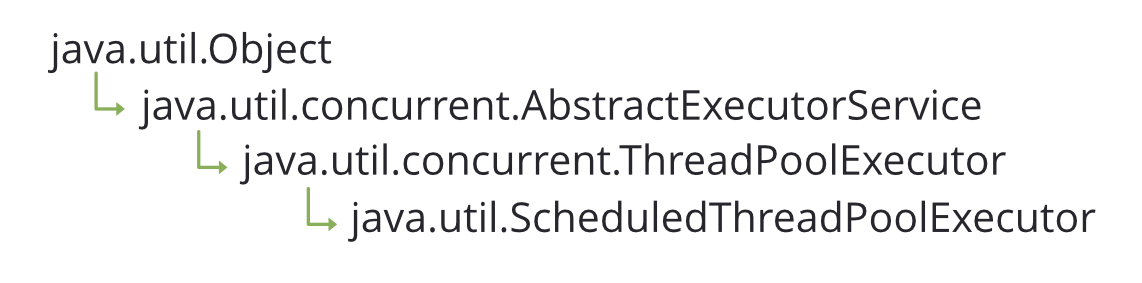GeeksforGeeks App
Open AppBrowser
Continue

## Related Articles

ScheduledThreadPoolExecutor class in Java is a subclass of ThreadPoolExecutor class defined in java.util.concurrent package. As it is clear from its name that this class is useful when we want to schedule tasks to run repeatedly or to run after a given delay for some future time.  It creates a fixed-sized Thread Pool. So when it is initiated, it needs to be given the corePoolSize (the number of threads in the Thread pool).

Class Hierarchy:Constructors:

• ScheduledThreadPoolExecutor(int corePoolSize) : Creates a new ScheduledThreadPoolExecutor object with the given pool size. It is to be noted that it creates a fixed-sized Thread Pool so once the corePoolSize is given, one can not increase the size of the Thread Pool.
• ScheduledThreadPoolExecutor(int corePoolSize, RejectedExecutionHandler handler): Creates a new ScheduledThreadPoolExecutor object with the given corePoolSize(ThreadPool size) and the handler that is used when the execution of a task is rejected (when the working queue is full or execution is blocked).

Besides these constructors, there is another way to get a ScheduledThreadPoolExecutor object. We can use Executors.newScheduledThreadPool(int corePoolSize) factory method defined by Executors class. It returns a ScheduledExecutorService object which can be type-casted to ScheduledThreadPoolExecutor object.

`ScheduledThreadPoolExecutor threadPool = (ScheduledThreadPoolExecutor)Executors.newScheduledThreadPool(4);`

Example 1:

## Java

 `// Java program to demonstrates ScheduleThreadPoolExecutor``// class``import` `java.util.*;``import` `java.util.concurrent.*;``import` `java.io.*;`` ` `class` `GFG {``    ``public` `static` `void` `main(String[] args)``    ``{`` ` `        ``// Creating a ScheduledThreadPoolExecutor object``        ``ScheduledThreadPoolExecutor threadPool``            ``= ``new` `ScheduledThreadPoolExecutor(``2``);`` ` `        ``// Creating two Runnable objects``        ``Runnable task1 = ``new` `Command(``"task1"``);``        ``Runnable task2 = ``new` `Command(``"task2"``);`` ` `        ``// Printing the current time in seconds``        ``System.out.println(``            ``"Current time : "``            ``+ Calendar.getInstance().get(Calendar.SECOND));`` ` `        ``// Scheduling the first task which will execute``        ``// after 2 seconds``        ``threadPool.schedule(task1, ``2``, TimeUnit.SECONDS);`` ` `        ``// Scheduling the second task which will execute``        ``// after 5 seconds``        ``threadPool.schedule(task2, ``5``, TimeUnit.SECONDS);`` ` `        ``// Remember to shut sown the Thread Pool``        ``threadPool.shutdown();``    ``}``}`` ` `// Class that implements the Runnable interface``class` `Command ``implements` `Runnable {``    ``String taskName;``    ``public` `Command(String taskName)``    ``{``        ``this``.taskName = taskName;``    ``}``    ``public` `void` `run()``    ``{``        ``System.out.println(``            ``"Task name : "` `+ ``this``.taskName + ``" Current time: "``            ``+ Calendar.getInstance().get(Calendar.SECOND));``    ``}``}`

Output :

```Current time : 51
```

Here the first task is executed after a two seconds delay and the second task is executed after the five seconds.

Example 2:

## Java

 `// Java program to demonstrates ScheduleThreadPoolExecutor``// class``import` `java.util.*;``import` `java.util.concurrent.*;``import` `java.io.*;`` ` `class` `GFG {``    ``public` `static` `void` `main(String[] args)``    ``{`` ` `        ``// Creating a ScheduledThreadPoolExecutor object``        ``ScheduledThreadPoolExecutor threadPool``            ``= ``new` `ScheduledThreadPoolExecutor(``2``);`` ` `        ``// Creating two Runnable objects``        ``Runnable task1 = ``new` `Command(``"task1"``);``        ``Runnable task2 = ``new` `Command(``"task2"``);`` ` `        ``// Printing the current time in seconds``        ``System.out.println(``            ``"Current time:"``            ``+ Calendar.getInstance().get(Calendar.SECOND));`` ` `        ``// Scheduling the first task which will execute``        ``// after 2 seconds and then repeats periodically with``        ``// a period of 8 seconds``        ``threadPool.scheduleAtFixedRate(task1, ``2``, ``8``,``                                       ``TimeUnit.SECONDS);`` ` `        ``// Scheduling the second task which will execute``        ``// after 5 seconds and then there will be a delay of``        ``// 5 seconds between the completion``        ``// of one execution and the commencement of the next``        ``// execution``        ``threadPool.scheduleWithFixedDelay(task2, ``5``, ``5``,``                                          ``TimeUnit.SECONDS);`` ` `        ``// Wait for 30 seconds``        ``try` `{``            ``Thread.sleep(``30000``);``        ``}``        ``catch` `(Exception e) {``            ``e.printStackTrace();``        ``}`` ` `        ``// Remember to shut sown the Thread Pool``        ``threadPool.shutdown();``    ``}``}`` ` `// Class that implements Runnable interface``class` `Command ``implements` `Runnable {``    ``String taskName;``    ``public` `Command(String taskName)``    ``{``        ``this``.taskName = taskName;``    ``}``    ``public` `void` `run()``    ``{``        ``try` `{``            ``System.out.println(``"Task name : "``                               ``+ ``this``.taskName``                               ``+ ``" Current time : "``                               ``+ Calendar.getInstance().get(``                                     ``Calendar.SECOND));``            ``Thread.sleep(``2000``);``            ``System.out.println(``"Executed : "` `+ ``this``.taskName``                               ``+ ``" Current time : "``                               ``+ Calendar.getInstance().get(``                                     ``Calendar.SECOND));``        ``}``        ``catch` `(Exception e) {``            ``e.printStackTrace();``        ``}``    ``}``}`

Output :

```Current time:26
Executed : task1 Current time : 30
Executed : task2 Current time : 33
Executed : task1 Current time : 38
Executed : task2 Current time : 40
Executed : task1 Current time : 46
Executed : task2 Current time : 47